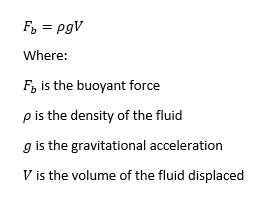# Help with Airship (LTA Vehicles) calculations and conversions

1. What do you want to achieve?
I am attempting to create realistic (as realistic as I can understand mathematically) airships, I.e. Zeppelins and Blimps. Do note that this is somewhat involved, but bear with me. If you know a lot of physics, feel free to comment on the formula I am using with any changes or suggestions (much appreciated). The primary question is converting formulas into studs, but stick around if you want to learn a little bit more about airships anyways.

In any case, I am currently calculating airship lift based on a few formulas. The first being:Things to note: Since the balloon that is (theoretically, as it is a simulation) filled with helium is lighter than air (LTA), it has lift acting upon it. This is Fb. p is the density of air, which I have a formula below for, g is 9.8 m/s or gravitational acceleration (note this is on Earth), and V is the volume displaced.

The calculation for air density is a little more involved. I am using the formula from here: atmospheric science - Air Density as a function of Altitude ONLY - Physics Stack Exchange,
as it already includes the density based upon average temperatures and more. The benefit of this function, is that the lift changes based on altitude (or y height), like it does for real blimps. This should make it so that the blimp reaches a point where it does not go higher.

Following this logic: https://kiss.caltech.edu/workshops/airships/presentations/hoffman.pdf, the helium ends up taking more space of the balloon the higher the airship goes. So, I can have the player control what percentage of the balloon is actually full, allowing the blimp to go up and down.

1. What is the issue?
I have attempted to make this as code in Lua, but to no avail. I seek advice on how to turn this into code, keeping in mind that the formulas are for real life and in meters, not studs. Additionally, how would I calculate the volume of a part assuming that its density is 0.0178 kg/m^3? (Heh, another case of conversion into studs).
2. What solutions have you tried so far?
I’ve attempted to do this a couple of times, most to no avail. I do believe this is stemming out of improper conversions, which is why I made this post. The developer forum does not have much on this.

The primary purpose of this post is so I can understand how to model this properly in Roblox. Entire scripts are not necessary, but any small samples or conversions of meters into studs are much appreciated. Also, how would you suggest I apply the calculated force to the balloon (Which currently is a mesh)? Bodymovers, etc.

Do note that I am only concerned with lift at the moment (up/down). Thrust comes later, after I can get this working.

Thank you for your time, and as well as any ideas you might have!

2 Likes

``````PhysicalProperties.new(Part.Material).Density
``````

According to this, 20 studs = 1m, thus gravity in RBX is 196.2 studs/s2

Calculating volume would be achievable through:

Vol = Mass / Density

``````local volume = Part:GetMass() / PhysicalProperties.new(Part.Material).Density
``````

However, you won’t be able to calculate the mass of the object if it’s a mesh - pretty sure it’s not entirely accurate when it’s a MeshPart and not a BasePart. To do it using the density provided, just convert it to studs where 20 studs = 1 m

In regard to applying the force, it depends on what method you’d like to use? You could either apply it through a BodyForce or BodyVelocity - depends on your application.

Hope I didn’t miss anything, struggled skimming through the post.

3 Likes